Display Accessibility Tools

##7-1 Shapes and Designs

The Problems of this Unit explore properties of polygons. Through work on tasks that require drawing, building, measuring, and reasoning about the size and shape of polygons, your student will learn:

• How to sort polygons into classes according to the number, size, and relationships of their sides and angles
• How to find angle measures by estimation, by use of tools like protractors and angle rulers, and by reasoning with variables and equations
• Formulas for finding the sum of the interior and exterior angles in any polygon
• The relationships of complementary and supplementary pairs of angles, such as those formed by interior and exterior angles of polygons, and in figures where parallel lines are cut by transversals
• How to apply and design angle-side measurement conditions needed for drawing triangles and quadrilaterals with specific properties
• The symmetry, tiling, and rigidity or flexibility properties of polygons that make them useful in buildings, tools, art and craft designs, and natural objects

As your child works on the Problems in this Unit, ask questions about situations that involve shapes such as:

• What do these polygons have in common? How do they differ from each other?
• When should I use estimation, freehand drawing, or special tools to measure and construct angles and polygons?
• How do the side lengths and angles of polygons determine their shapes?
• Why do certain polygons appear so often in buildings, artistic designs, and natural objects?
• How can I give directions for constructing polygons that meet conditions of any given problem?

##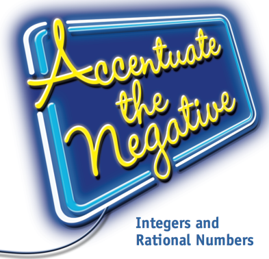7-2 Accentuate the Negative

In Accentuate the Negative, your student(s) will extend their knowledge of negative numbers. They will use negative numbers to solve problems. They will learn how to:

• Use appropriate notation to indicate positive and negative numbers and zero
• Compare and order rational numbers and locate them on a number line
• Understand the relationship between a number and its opposite (additive inverse)
• Relate direction and distance to the number line
• Develop and use different models (number line, chip model) for representing addition, subtraction, multiplication, and division
• Develop algorithms for adding, subtracting, multiplying, and dividing positive and negative numbers
• Interpret and write mathematical sentences to show relationships and solve problems
• Write and use related fact families for addition/subtraction and multiplication/division to solve simple equations
• Use parentheses and the Order of Operations in computations
• Use the commutative properties of addition and multiplication
• Apply the Distributive Property to simplify expressions and solve problems
• Use models and rational numbers to represent and solve problems

When your child encounters a new problem, it is a good idea to ask questions such as:

• How do negative and positive numbers and zero help describe the situation?
• What will addition, subtraction, multiplication, or division of rational numbers tell about the problem?
• What model(s) for positive and negative numbers and zero help show relationships in the problem situation?

##7-3 Stretching and Shrinking

In Stretching and Shrinking, your child will learn the mathematical meaning of similarity, explore the properties of similar figures, and use similarity to solve problems. Your student will learn how to:

• Identify similar figures by comparing corresponding sides and angles
• Use scale factors and ratios to describe relationships among the side lengths, perimeters, and areas of similar figures
• Construct similar figures (scale drawings) using informal methods, scale factors, and geometric tools
• Use algebraic rules to produce similar figures and recognize when a rule shrinks or enlarges a figure
• Predict the ways that stretching or shrinking a figure will affect side lengths, angle measures, perimeters, and areas
• Use the properties of similarity to find distances and heights that cannot be measured directly
• Use scale factors or ratios to find missing side lengths in a pair of similar figures

When your child encounters a new problem, it is a good idea to ask questions such as:

• What determines whether two shapes are similar?
• What is the same and what is different about two similar figures?
• When figures are similar, how are the side lengths, areas, and scale factors related?
• How can I use similar figures to find missing measurements?

##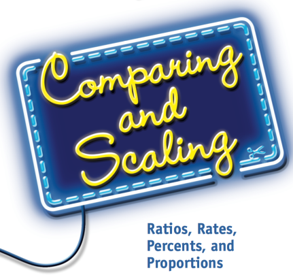7-4 Comparing and Scaling

In this Unit, your student(s) will extend their knowledge of ratios, proportions, and proportional reasoning. They will learn how to:

• Analyze comparison statements for correctness and quality;
• Use ratios, rates, and percents to write comparison statements;
• Distinguish between and use part-to-part and part-to-whole ratios to make comparisons;
• Scale a ratio, rate, or percent to solve a problem;
• Set up and solve proportions;
• Find unit rates and use them to solve problems;
• Recognize proportional situations from a table, graph, or equation;
• Connect a unit rate and a constant of proportionality to a table, graph, or equation representing a situation.

As you work on the Problems in this Unit, ask yourself these questions about situations that involve comparisons.

• What quantities are being compared?
• Why does the situation involve a proportional relationship (or not)?
• How might ratios, rates, or a proportion be used to solve the problem?

##7-5 Moving Straight Ahead

In Moving Straight Ahead, your child will explore properties of linear relationships and linear equations, and will learn how to:

• Recognize problem situations that involve linear relationships
• Construct tables, graphs, and symbolic equations that represent linear relationships
• Translate information about linear relations given in a verbal description, a table, a graph, or an equation to one of the other forms
• Connect equations that represent linear relationships to the patterns in tables and graphs of those equations
• Identify the rate of change, slope, and y-intercept from the graph of a linear relationship
• Solve linear equations
• Write and interpret equivalent expressions as well as determine whether two or more expressions are equivalent
• Solve problems and make decisions about linear relationships using information given in tables, graphs, and equations
• Solve problems that can be modeled with inequalities and graph the solution set

When your child encounters a new problem, it is a good idea to ask questions such as:

• What are the variables in the problem?
• Do the variables in the problem have a linear relationship to each other?
• What patterns in the problem suggest that the relationship is linear?
• How can the linear relationship in a situation be represented with a verbal description, a table, a graph, or an equation?
• How do changes in one variable affect changes in a related variable?
• How are these changes captured in a table, a graph, or an equation?
• How can tables, graphs, and equations of linear relationships be used to answer questions?

##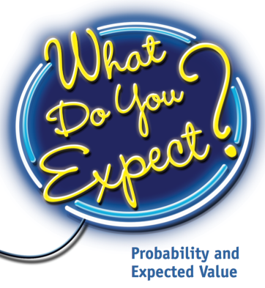7-6 What Do You Expect?

In this Unit, your child will deepen their understanding of basic probability concepts and will learn about the expected value of situations involving chance. They will learn how to :

• Use probabilities to predict what will happen over the long run
• Distinguish between equally likely events and those that are not equally likely
• Use strategies for identifying possible outcomes and analyzing probabilities, such as using lists or tree diagrams
• Develop two kinds of probability models:
• Gather data from experiments (experimental probability)
• Analyze possible outcomes (theoretical probability)
• Understand that experimental probabilities are better estimates of theoretical probabilities when they are based on larger numbers of trials
• Determine if a game is fair or unfair
• Use models to analyze situations that involve two stages (or actions)
• Determine the expected value of a chance situation
• Analyze situations that involve binomial outcomes
• Interpret statements of probability to make decisions and answer questions

As you work on Problems in this Unit, ask your child questions about situations that involve analyzing probabilities:

• What are the possible outcomes for the event(s) in this situation?
• Are these outcomes equally likely?
• Is this a fair or unfair situation?
• Can I compute the theoretical probabilities or do I conduct an experiment?
• How can I determine the probability of one event followed by a second event: two-stage probabilities?
• How can I use expected value to help me make decisions?

##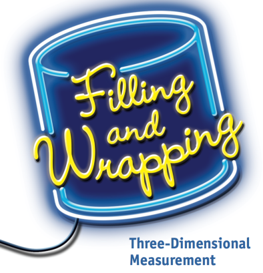7-7 Filling and Wrapping

In Filling and Wrapping, your child will explore surface area and volume of three-dimensional objects, and will learn how to:

• Develop the ability to visualize and draw three-dimensional shapes
• Develop strategies for finding volumes of three-dimensional objects, including prisms, cylinders, pyramids, cones, and spheres
• Design and use nets to develop formulas for finding surface areas of prisms and cylinders
• Develop formulas and strategies for finding the area and circumference of a circle
• Explore patterns relating the volumes of prisms, cylinders, cones, spheres, and pyramids
• Understand that three-dimensional figures may have the same volume but different surface areas
• Investigate the effects of scaling dimensions of figures on the volume and surface area
• Recognize and solve problems involving volume and surface area

When your child encounters a new problem, it is a good idea to ask questions such as:

• What are the shapes and properties of the figures in the problem?
• Which measures of a figure are involved—length, surface area, or volume?
• What measurement strategies or formulas might help in using given information to find unknown measurements?

##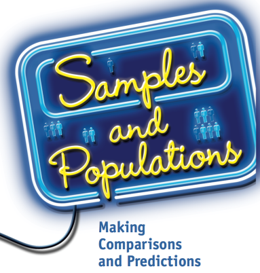7-8 Samples and Populations

In Samples and Populations, your student(s) will learn about different ways to collect and analyze data in order to make comparisons and draw conclusions. They will learn how to:

• What is the population?
• What is the sample?
• Is the sample a representative sample?
• How can I describe the data I collected?
• How can I use my results to draw conclusions about the population?
• How can I use samples to compare two or more populations?